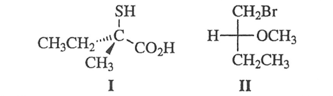Problem: What are the absolute configurations of these two molecules? a) S(I) and S(II) b) S(I) and R(II) c) R(I) and S(II) d) R(I) and R(II)

100% (3 ratings)
Problem Details

What are the absolute configurations of these two molecules?

a) S(I) and S(II)

b) S(I) and R(II)

c) R(I) and S(II)

d) R(I) and R(II)